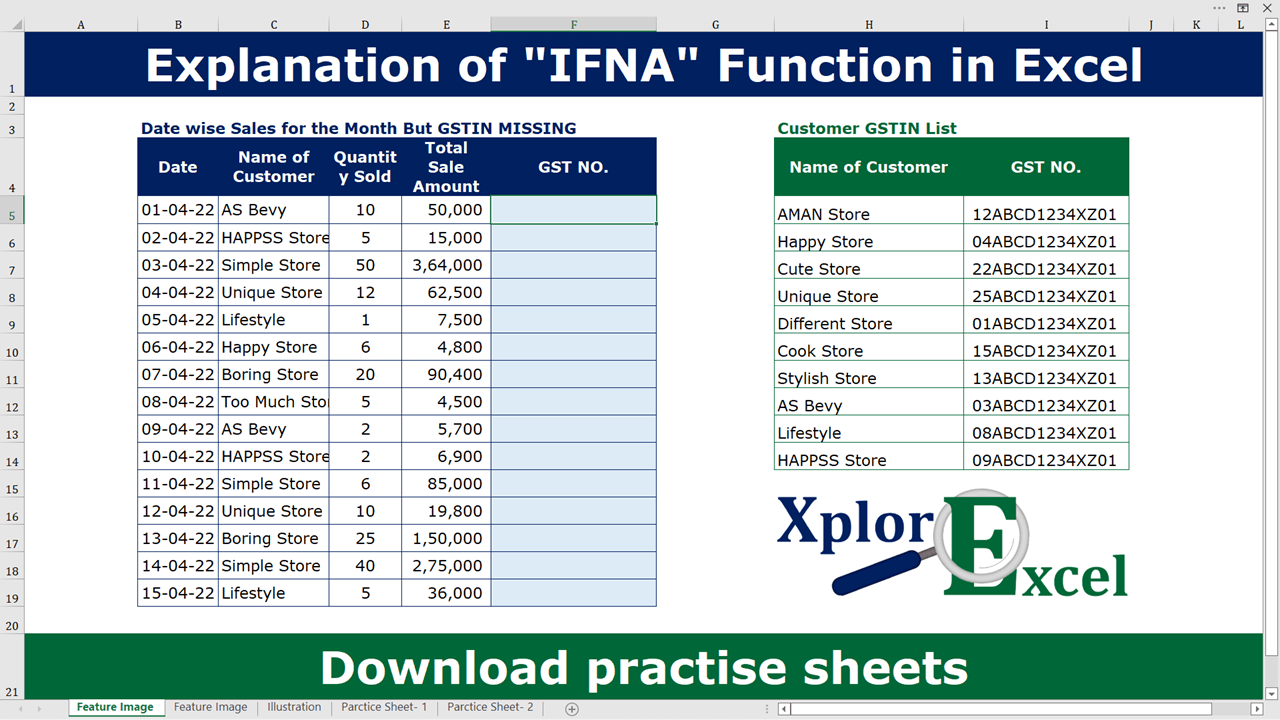# IFNA Excel Function | Free 2 Practice workbooksExplanation of IFNA Function in Excel-min

In this article, I have explained to you, how to use the IFNA Excel Function in Excel with other Excel Formulas and Functions Like, IFNA with VLOOKUP and IFNA with HLOOKUP. So let’s start.

## IFNA Excel Function

The IFNA Excel Function can use with other excel functions. where other functions or formulas of excel can not find any value as a result then It shows the already given sentence (i.e. Not found) instead of an error of #NA.

It works like the IFERROR excel function but it is worked only with the error of #N/A and ignore all other logical and human errors. So in the case where you only want to replace an #N/A error with specific text then you can use the IFNA excel function.

We can use this function where we already know that the function/formula will generate an error of #N/A in some cases. Suppose, if you want to show some content from another workbook to your current workbook with the help of the VLOOKUP formula of excel then it is possible that there will be something that is not available in the previous workbook, So VLOOKUP will show an error of #N/A, which make your workbook look ugly. So with the help of the IFNA formula of Excel, you can replace the error word(i.e. #N/A) with the words you want(i.e. Not Found).

IFNA is the narrow version of the IFERROR formula. It is just a specific function that works with only a single error i.e. #N/A. We can use both formulas in place of each other.

### The feature of the “IFNA” function

• IFNA will find results until it got an error.
• With the help of the IFNA Function, we can make our project neet and clear by replacing errors with predefined text or Blank Cell.
• It will removed/Replaced excel error of #N/A.
• It can be used with all other fucntions of excel which can generate an error of #N/A.

## Explanation of IFNA Excel Function

Now, We will explain the Arguments of the Function.

=IFNA(value, value_if_error)

value:- Select the value on which you want to apply the error rule. You can select the cell or write the already applied formula here.

value_if_error: – You have to write that value which you want to show if there is an error in the applied function. it may be Not found, New, no, 1,0, blank, etc. whatever you want to show.

Following errors are evaluated by this formula:
#N/A,

## Example of Explanation of IFNA Excel Function: –

In the following table, we want to retrieve the result of the GST Numbers without an error of the #N/A message. The table of date-wise sales is given in the workbook.

### Solution:

We will Apply the IFNA function and get the desired result. This is shown in the following steps: –

#### Step No. 1

We will Copy the Vlookup formula from cell F4 and paste it in the H17. Then clear the all cell containing Vlookup.

#### Step No. 2

We will write the “=IFNA( ” in the column of the result

#### Step No. 3:

Now select the value(function) from which you want to check the errors. Like I am coping already applied formula of VLOOKUP in column E and paste it in the value part of IFNA Function.

you can also write the new formula or any formula for calculation that you are using while calculating something.

#### Step No. 4

Now please select the value_if_error that will be shown when there is an error in the selected function.

I have just written “New Customer”. You can write any message here but remember one thing if you want to use text then use it within inverted commas like “your text” or if you want to show Number then you can write it without inverted commas.

#### Step No. 5

Now, Clear the cell H17 because don’t need it more.

#### Step No. 6

Now, copy the newly created formula from cell G4 and paste it into the remaining cell to check out the error from the all given data.

Now we got our result as desired.

## Practice IFNA Function online:

This is all about the IFNA function in excel.

Thanks, If you have any confusion in this formula please write a comment in the comment box we reply to you as soon as possible.